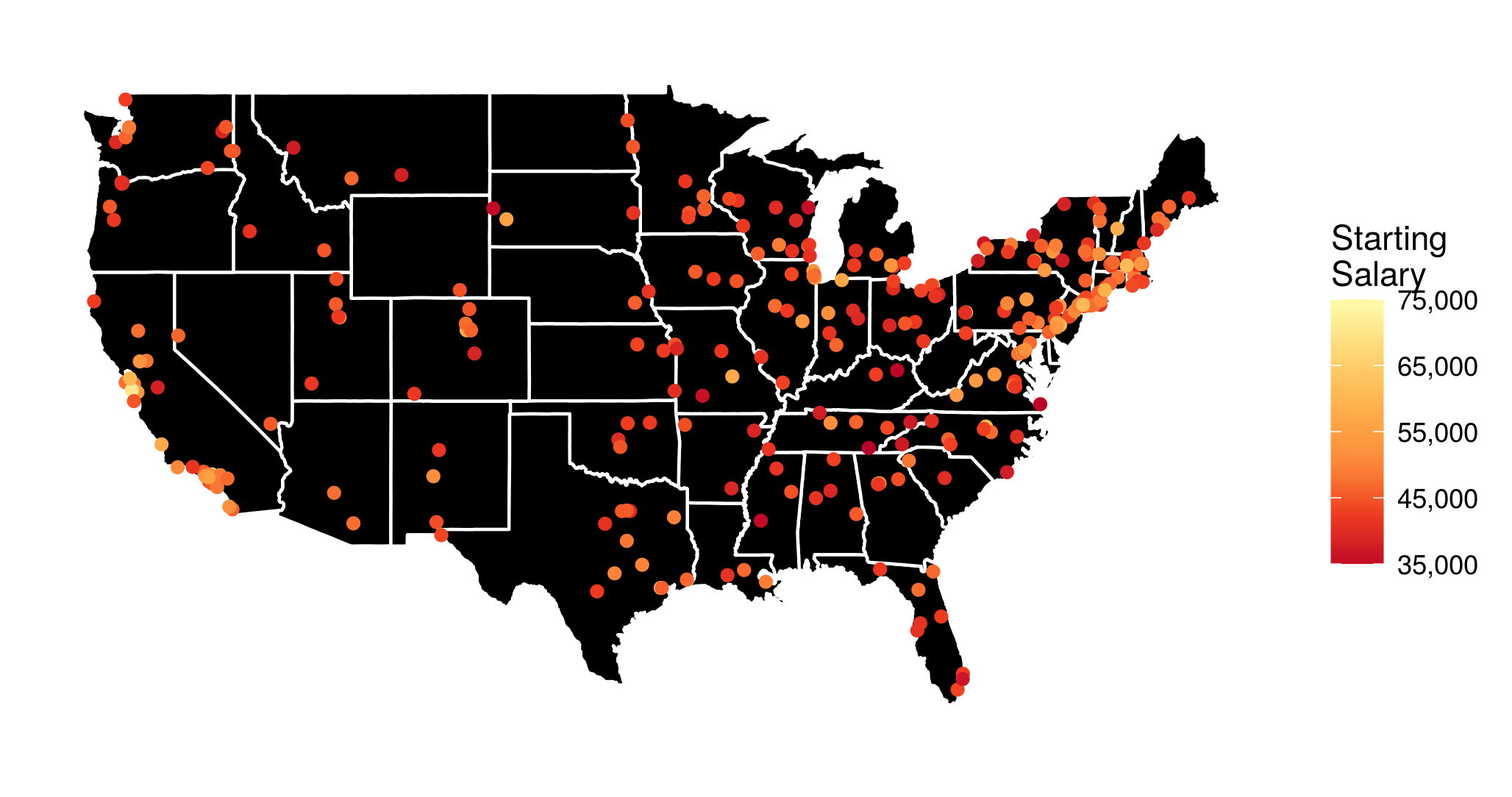Based on an interesting dataset from the Wall Street Journal I made the above visualization of the median starting salary for US college graduates from different undergraduate institutions (I have also looked at the mid-career salaries, and the salary increase, but more on that later). However, I thought that it would be a lot more informative, if it were interactive. To the very least I wanted to be able to see the school names when hovering over or clicking on the points with the mouse.

Luckily, this kind of interactivity can be easily achieved in R with the library plotly, especially due to its excellent integration with ggplot2, which I used to produce the above figure. In the following I describe how exactly this can be done.

Before I show you the interactive visualizations, a few words on the data preprocessing, and on how the map and the points are plotted with ggplot2:

• I generally use functions from the tidyverse R packages.
• I save the data in the data frame salaries, and transform the given amounts to proper floating point numbers, stripping the dollar signs and extra whitespaces.
• The data provide school names. However, I need to find out the exact geographical coordinates of each school to put it on the map. This can be done in a very convenient way, by using the geocode function from the ggmap R package:
school_longlat <- geocode(salaries$school) school_longlat$school <- salaries$school salaries <- left_join(salaries, school_longlat)  • For the visualization I want to disregard the colleges in Alaska and Hawaii to avoid shrinking the rest of the map. The respective rows of salaries can be easily determined with a grep search: grep("alaska", salaries$school, ignore.case = 1)
#  206
grep("hawaii", salaries\$school, ignore.case = 1)
#  226

• A data frame containing geographical data that can be used to plot the outline of all US states can be loaded using the function map_data from the ggplot2 package:
states <- map_data("state")

• And I load a yellow-orange-red palette with the function brewer.pal from the RColorBrewer library, to use as a scale for the salary amounts:
yor_col <- brewer.pal(6, "YlOrRd")

• Finally the (yet non-interactive) visualization is created with ggplot2:
p <- ggplot(salaries[-c(206, 226), ]) +
geom_polygon(aes(x = long, y = lat, group = group),
data = states, fill = "black",
color = "white") +
geom_point(aes(x = lon, y = lat,
color = starting, text = school)) +
coord_fixed(1.3) +
colors = rev(yor_col),
labels = comma) +
guides(size = FALSE) +
theme_bw() +
theme(axis.text = element_blank(),
axis.line = element_blank(),
axis.ticks = element_blank(),
panel.border = element_blank(),
panel.grid = element_blank(),
axis.title = element_blank())


Now, entering p into the R console will generate the figure shown at the top of this post.

However, we want to…

## …make it interactive

The function ggplotly immediately generates a plotly interactive visualization from a ggplot object. It’s that simple!(Though I must admit that, more often than I would be okay with, some elements of the ggplot visualization disappear or don’t look as expected.)

The function argument tooltip can be used to specify which aesthetic mappings from the ggplot call should be shown in the tooltip. So, the code

ggplotly(p, tooltip = c("text", "starting"),
width = 800, height = 500)


generates the following interactive visualization.

Now, if you want to publish a plotly visualization to https://plot.ly/, you first need to communicate your account info to the plotly R package:

Sys.setenv("plotly_username" = "??????")
Sys.setenv("plotly_api_key" = "????????????")


and after that, posting the visualization to your account at https://plot.ly/ is as simple as:

plotly_POST(filename = "Starting", sharing = "public")


## More visualizations

Finally, based on the same dataset I have generated an interactive visualization of the median mid-career salaries by undergraduate alma mater (the R script is almost identical to the one described above). The resulting interactive visualization is embedded below.

Additionally, it is quite informative to look at a visualization of the salary increase from starting to mid-career.